# Motion problems + system of equations - math problems

#### Number of problems found: 68

• The train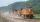The train passed the observer in the 20s and passed through a 1500m tunnel in 2 minutes. Calculate the speed (km/h) and train length.
• Tractor wheels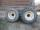Smaller tractor wheel has a circumference of 3 meters, bigger 4 meters. On what path executes smaller wheel 6 turns over more bigger?
• Water current speed.Two cities along the river are 100 km apart. The powerboat downstream runs for 4 hours, upstream for 10 hours. Determines the river's current speed.
• Distance between two citiesCar went from A to B 4h. On the way back, the car was up 15km / h faster. The return trip took 48 minutes. Shorter than the way there. Find the distance of the cities A and B.
• Cyclist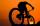Cyclist rode two-fifths of the way the first day. The second day drove 5 km less while traveled three-eighths of route. How many kilometers does he have to finish target?
• Car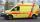Car goes some distance in 3 hours and 20 minutes. If it increase speed by 10 km/h, i goes this distance in 2.5 hours. Calculate the distance.
• MotionFrom two different locations distant 232 km started against car and bus. The car started at 5:20 with average speed 64 km/h. Bus started at 7:30 with average speed 80 km/h. When they meet? How many kilometers went the bus?
• Truck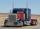Truck went for 4 hours and 30 minutes. If truck increase speed by 11 km/h, went same distance in 2 hours. Calculate this distance.
• Speed of car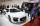The car went to a city that was 240 km away. If his speed increased by 8 km/h, it would reach the finish one hour earlier. Determine its original speed.
• Two trucksTwo trucks left cities A and B against each other and met after an hour. The first car came to B 27 minutes later than the second car to A. Calculate the car speed if the distance between cities A, B is 90 km.
• Motorbike circuit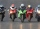On a 2,550 m long circuit, two motorcycles go at such speeds that they meet every minute if they go against each other and run every 5 minutes if they go in the same direction. Find their speeds.
• The hikerThe hiker will travel 40% of the route on the first day 1and/3 of the rest od second day. Last day 30 km. What was the length of the 3-day trip? How many kilometers did he walk each day?
• The carThe car has traveled the distance between A and B for four hour. If we increased the average by 17 km/h the car travel this distance an hour earlier. Determine the initial speed of the car and the distance between A and B.
• Motion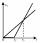The two bodies move in the same direction evenly in a straight line, at speeds of 5 cm/s and 10 cm/s. The movement of the first body started 2 seconds earlier than the movement of the second body, from a point located at a distance of 20 cm from the start
• Scouts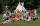The boys from scout group traveled 5 days distance 115 km. Every day walked 1.5 km less than the previous day. How many kilometers scouts walked in the first day?
• Walkers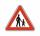From points A and B simultaneously started against each other two walkers. After meeting both continue to B. Second walker came to B 2 hours before the first walker. It's speed is 2.7 times of speed of the first pedestrian. How many hours went pedestrians
• Car and motorcyclistA car and a motorcyclist rode against each other from a distance of 190 km. The car drove 10km/h higher than the motorcyclist and started half an hour later. It met a motorcyclist in an hour and thirty minutes. Determine their speeds.
• Three-day tripThe cyclist on three-day trip travel 30% of the total route on the first day, 3/5 of the rest on the second day and 35 km on the third day. How many kilometers did travel cyclists each day and how many?
• Scouts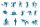Three scouts went on a three day trip. The second day went 4 km more than the first day. The third day went two times less than the first day. They went along 54 km. How many kilometers went every day together?
• The touristThe tourist traveled 190km in 5 hours. Part of the journey passed at 5 km/h. The rest he went by bus at a speed of 60 km/h. How long has a bus gone?

Do you have an interesting mathematical word problem that you can't solve it? Submit a math problem, and we can try to solve it.

We will send a solution to your e-mail address. Solved examples are also published here. Please enter the e-mail correctly and check whether you don't have a full mailbox.

Please do not submit problems from current active competitions such as Mathematical Olympiad, correspondence seminars etc...

Do you have a system of equations and looking for calculator system of linear equations? Motion problems - math word problems. System of equations - math word problems.